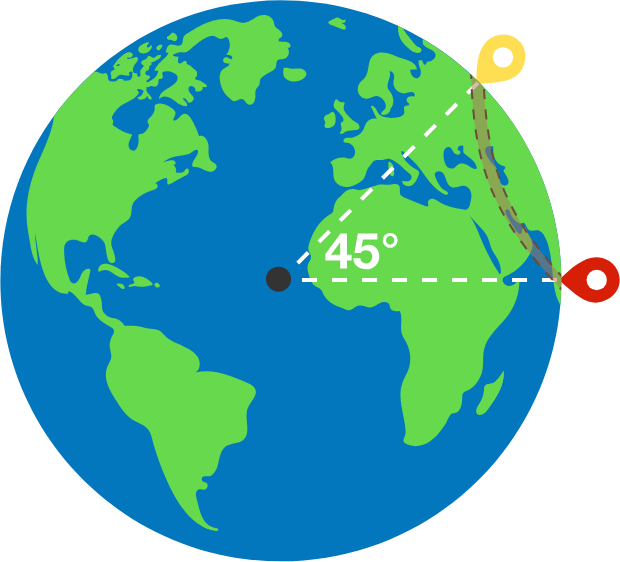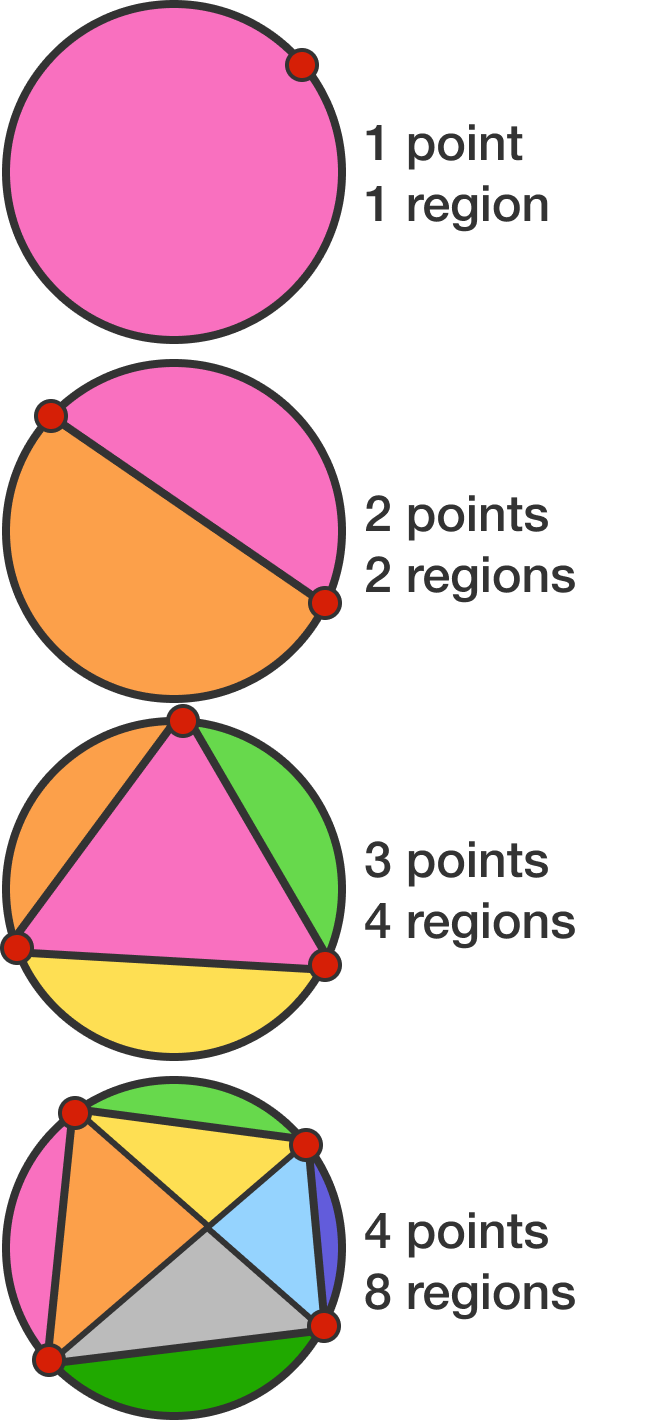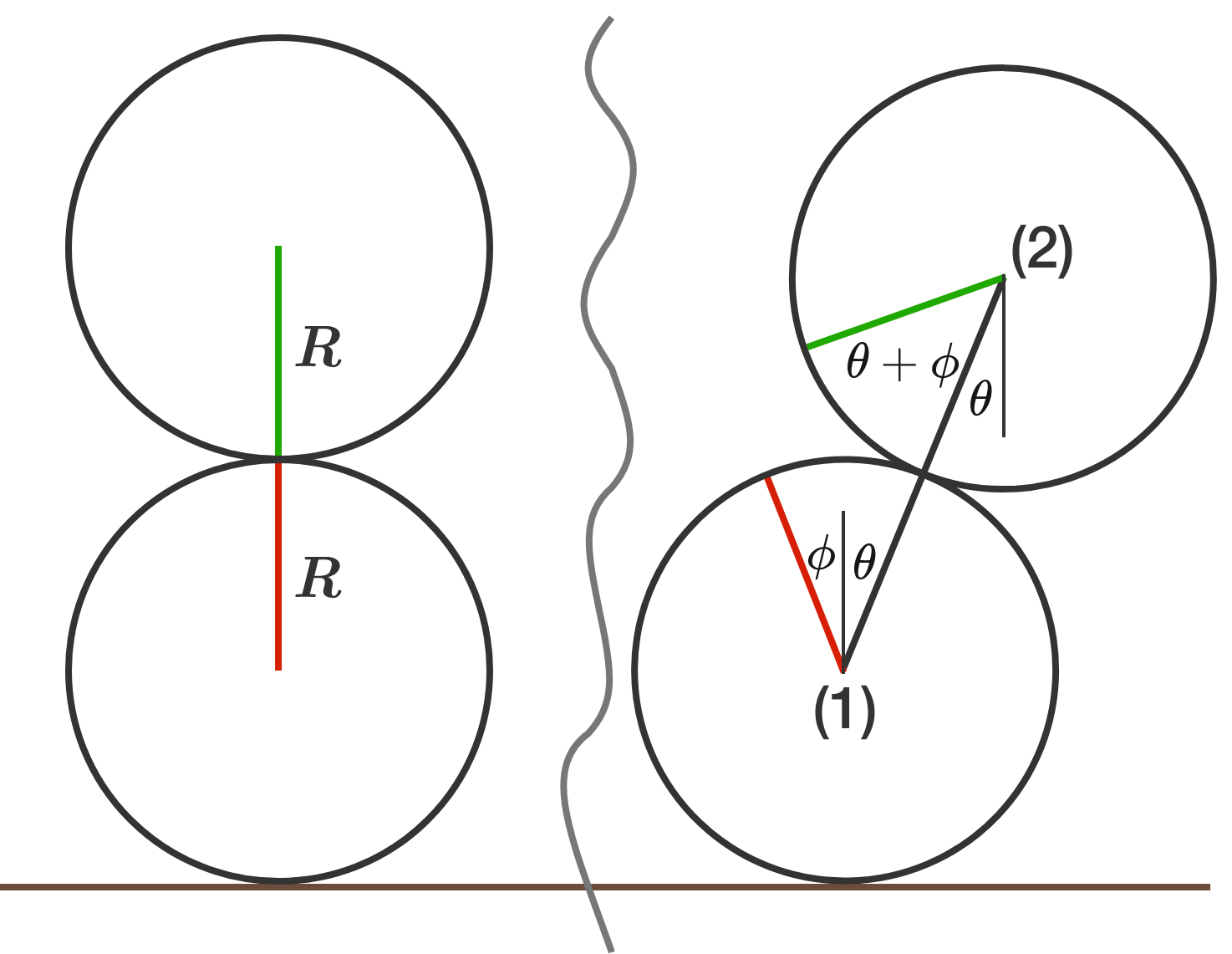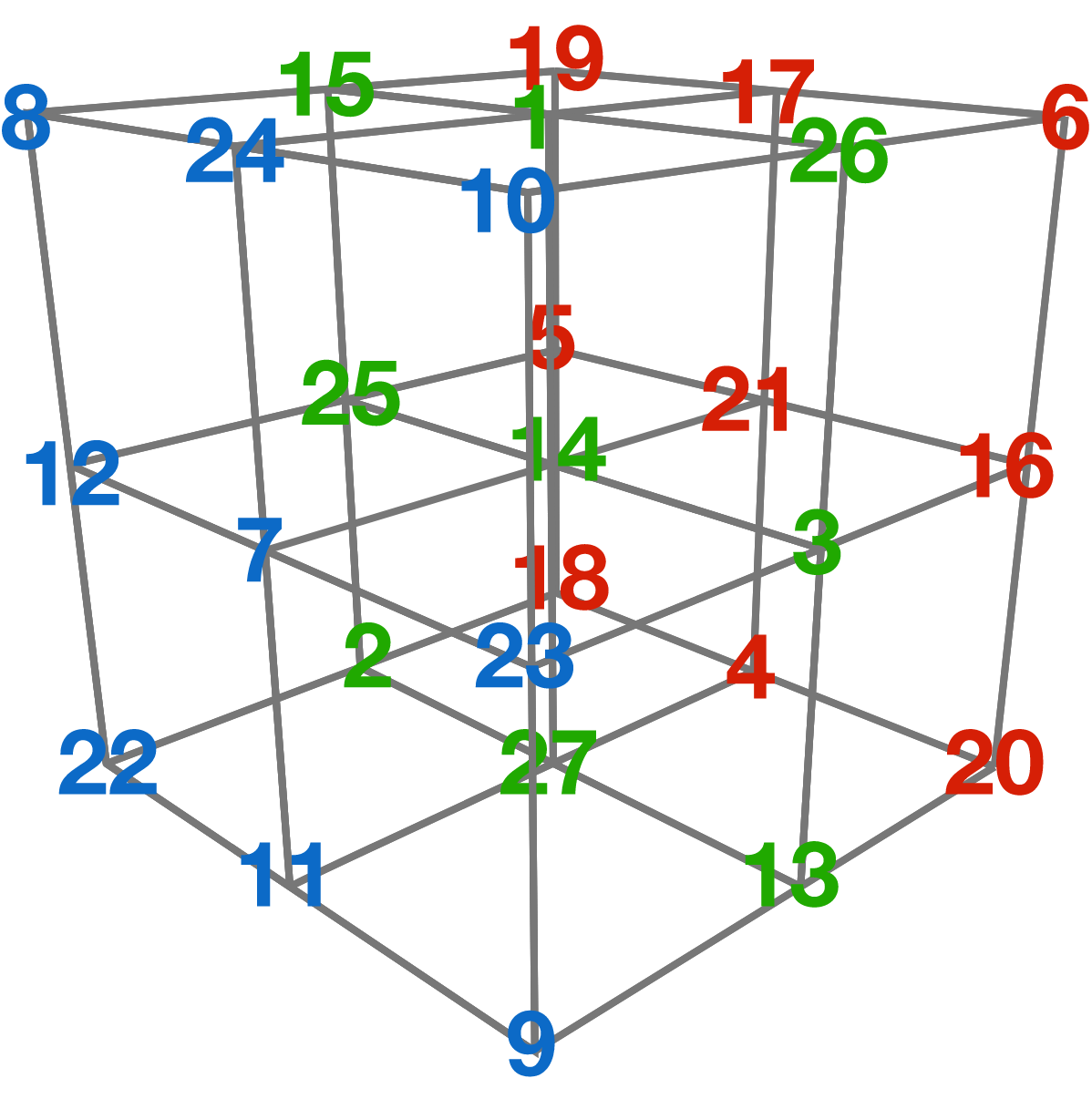# Problems of the Week

Contribute a problemTwo cities are situated at an angular distance of $45^\circ$ with respect to Earth's center.

You are asked to design a tunnel through Earth’s crust connecting the two cities such that it minimizes the time $T$ to commute between the cities when the train moves only under the influence of gravity.

What is this minimum $T?$

Details and Assumptions:

• Assume Earth to be a sphere of uniform mass density.
• $R$ is the radius of Earth.
• $g$ is acceleration due to gravity.
• Ignore friction.Select a number of points along the circumference of a circle and connect each pair with a chord. These chords will divide the interior of the circle into a number of regions.

The maximum numbers of regions are shown at right for 1 to 4 points.

What is the maximum number of regions for six points?

Imagine you arrange the thirteen integers $1$ to $13$ in a row and label them $a_1, a_2, \ldots, a_{13}$ from left to right under the following conditions:

• $a_{n+2} > a_n$ for $n = 1, 2, \ldots, 11.$
• $a_n$ is either $n - 1, n,$ or $n + 1$ for all $n=1, 2, \ldots, 13.$

How many such arrangements are possible?

Inspiration

A solid cylinder of radius $R$ rests on another identical cylinder that rests on the floor in an unstable equilibrium.

If the system is slightly disrupted and there's no sliding between any surfaces, with the cylinders maintaining contact, the angle $\theta$ that the line joining the centers make with the vertical satisfies $\dot{\theta}^2=\frac{ag(1-\cos\theta)}{R\big(b+c\cos\theta-d\cos^2\theta\big)},$ where $g$ is the acceleration due to gravity and $a, b, c, d$ are positive integers such that the fraction is irreducible.

What is $a+b+c+d?$A $3 \times 3 \times 3$ magic cube is a three-dimensional array of the consecutive integers $1$ through $27,$ with the special property that the sum along any row, any column, any pillar, or any of the four space diagonals is equal to the same number.

How many different $3 \times 3 \times 3$ magic cubes are there?

Note: Rotations of a certain solution are considered the same solution and therefore not counted.

×